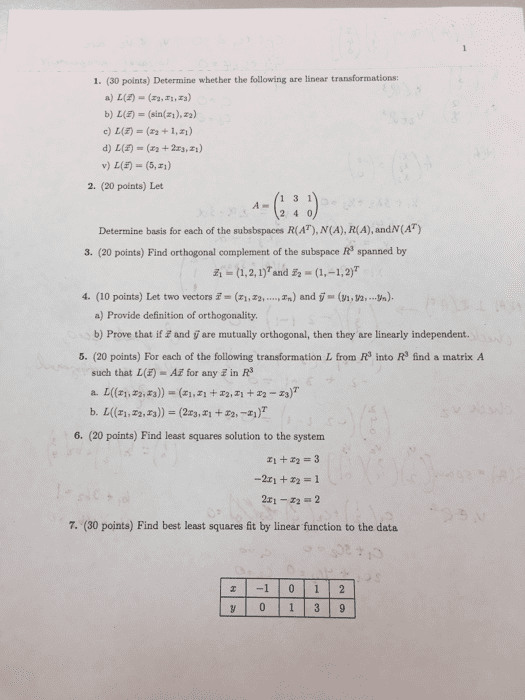1
0
watching
215
views11 Nov 2019

# Test review1. (30 polnts) Determine whether the following are linear transformations: a) L(å¸-(n,n,n) b) L(E) (sin(),2) v) L(F) = (5 ) 2. (20 points) Let 2 4 0 Determine basis for each of the subsbspaces R(AT), N(A), R(A), andN(AT) 3. (20 points) Find orthogonal complement of the subspace R3 spanned by Â¡Â¡ = (1, 2, 1)Tand -(1,-1,2)T (10 points) Let two vectors i. (zi,z2, a) Provide definition of orthogonality b) Prove that if and y are mutually orthogonal, then they are linearly independent. 4. , zn) ard v_ (n,n, %). 5. (20 points) For each of the following transformation L from R3 into R3 find a matrix A such that L(E)A for any in R 6. (20 points) Find least squares solution to the system 1+2 3 -2x1 +x2 = 1 2x1-2 2 7. (30 points) Find best least squares ft by linear function to the data